# For the structure and loading of Prob. 2.48, determine (a) the value of α for which the tension in cable BC is as small as possible, (b) the corresponding value of the tension.

Question-AnswerCategory: Engineering MechanicsFor the structure and loading of Prob. 2.48, determine (a) the value of α for which the tension in cable BC is as small as possible, (b) the corresponding value of the tension.

For the structure and loading of Prob. 2.48, determine (a) the value of α for which the tension in cable BC is as small as possible, (b) the corresponding value of the tension.Step: 1

a)
A force triangle is drawn to show the system in equilibrium.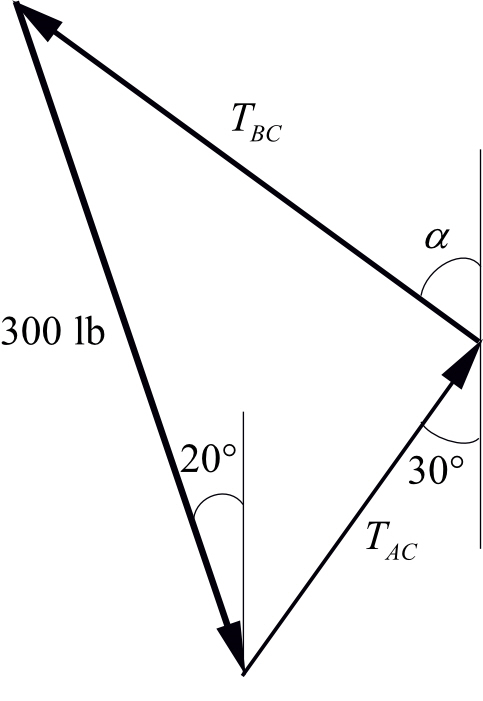Step: 2

Apply the law of sines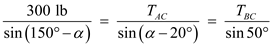Consider the following relation as shown below.…… (1)
The tension in the cablewill be minimum, if the value of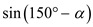is maximum.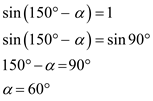Therefore, the tension in the cablewill be minimum, when.

Step: 3

b)
Tension in the cable BC can be found by substituting 60o forin equation (1).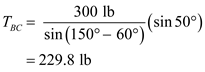Therefore, the tension in the cable BC is.